# Variables & Data structures

## Variables

We use the keyword var to define a variable. The variable type comes after the variable name.

``````// define a variable with name "numberOfTimes" and type "int"
// the examples are of int, but you can use any other data type.
var numberOfTimes int

// define three variables which types are "int"
var var1, var2, var3 int

// define a variable with name "numberOfTimes”, type "int"
// and value "3"
var numberOfTimes int = 3

/*
Define three variables with type "int", and
initialize their values.
var1 is 1, var2 is 2, var3 is 3.
*/
var var1, var2, var3 int = 1, 2, 3
``````

#### Variable declaration Shortcut

``````/*
Define three variables without type "type" and
without keyword "var", and initialize their values.
vname1 is v1，vname2 is v2，vname3 is v3
*/
vname1, vname2, vname3 := v1, v2, v3
``````

`:=` can only be used inside functions, for defining global variables we have to stick to using var. It throws a syntax error otherwise.

#### Blank variable

`_` is called the blank variable and it is used to ignore a value.

Suppose we have a function divide, which takes two arguments, and will return the quotient and remainder of arg1 divided by arg2. But we only want the remainder, so we ignore the quotient using

``````    _ , remainder := divide(10, 3)
``````

Unused variables cause compilation errors. Compile the following code and see what happens. Sometimes, we use this notations to import libraries which we do not want to use as prefix, while working with databases we do not want to prefix the library name to each function call of the library, so we use the blank variable. We will see that in a future chapter.

``````package main

func main() {
var i int
}
``````

## Constants

Constants are the values that are determined during compile time that cannot be changed during runtime. In Go, you can use number, boolean or string as types of constants.

#### Defining constants

``````const constantName = value
// you can assign type of constants if it's necessary
const Pi float32 = 3.1415926
``````

More examples.

``````const Pi = 3.1415926
const i = 10000
const prefix = "astaxie_"
``````

## Elementary types

#### Boolean

The keyword `bool` is used to define a variable of the boolean type. There are two boolean values, `true` or `false`; `false` will be the default value. Unlike other languages, Go doesn't allow us to convert boolean into number.

``````// sample code
var isActive bool  // global variable
var enabled, disabled = true, false  // omit type of variables
func test() {
var available bool  // local variable
valid := false      // brief statement of variable
available = true    // assign value to variable
}
``````

#### Numerical types

###### Integers

Integer types include signed and unsigned integer types, `int` and `uint` respectively. They have the same length, but the specific length depends on your operating system. They use 32-bit in 32-bit operating systems, and 64-bit in 64-bit operating systems. Go also has types that have specific length including `rune`, `int8`, `int16`, `int32`, `int64`, `byte`, `uint8`, `uint16`, `uint32`, `uint64`. Note that `rune` is alias of `int32` and `byte` is alias of `uint8`.

Go doesn't allow assigning values between data types. The following operation will cause compilation errors.

``````var a int8

var b int32

c := a + b
``````

Although int32 has a longer length than int8, and has the same type as int, you cannot assign values between them. ( c will be asserted as type `int` here )

###### Fractions

Float types have the `float32` and `float64` types and no type called `float`. The latter one is the default type if using brief statement.

###### Complex numbers

Go supports complex numbers as well. `complex128` (with a 64-bit real and 64-bit imaginary part) is the default type, if you need a smaller type, there is one called `complex64` (with a 32-bit real and 32-bit imaginary part). Its form is `RE+IMi`, where `RE` is real part and `IM` is imaginary part, the last `i` is the imaginary number. There is a example of complex number.

``````    var c complex64 = 5+5i
//output: (5+5i)
fmt.Printf("Value is: %v", c)
``````

#### String

Go uses the UTF-8 character set. Strings are represented by double quotes `""` or backticks ``` `.

``````// sample code
var frenchHello string  // basic form to define string
var emptyString string = ""  // define a string with empty string
func test() {
no, yes, maybe := "no", "yes", "maybe"  // brief statement
japaneseHello := "Ohaiou"
frenchHello = "Bonjour"  // basic form of assign values
}
``````

String objects do not now allow value change. You will get errors when you compile the following code.

``````var s string = "hello"
s = 'c'
``````

For changing a string, a new string has to be created using the old string. Go doesn't allow modifications to a string variable, but you can always create a new one.

``````s := "hello"
c := []byte(s)  // convert string to []byte type
c = 'c'
s2 := string(c)  // convert back to string type
fmt.Printf("%s\n", s2)
``````

`+` operator can be used to concatenate two strings.

``````s := "hello,"
m := " world"
a := s + m
fmt.Printf("%s\n", a)
``````

and also.

``````s := "hello"
s = "c" + s[1:] // returns substring from index 1 till the end.
fmt.Printf("%s\n", s)
``````

` (backtick) is used to have a multiple-line string.

``````m := `hello
world`
``````

\ ` (backtick) will not escape any characters in a string.

#### Error types

Go doesn't have the try catch block. There is one `error` type for the purpose of dealing with errors in the package called `errors`. Go requires us to explicitly handle our errors or ignore them. Typical error handling forms a `if err != nil` ladder.

``````err := errors.New("emit macho dwarf: elf header corrupted")
if err != nil {
fmt.Print(err)
}
``````

## Underlying data structures

The following picture comes from an article about Go data structure in Russ Cox's Blog. As you can see, Go utilizes blocks of memory to store data.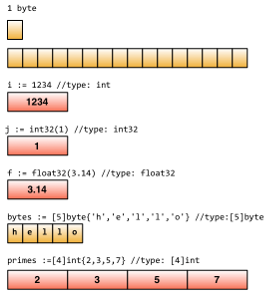## Important points

#### Define by group

If you want to define multiple constants, variables or import packages, you can use the group form.

Basic form.

``````import "fmt"
import "os"

const i = 100
const pi = 3.1415
const prefix = "Go_"

var i int
var pi float32
var prefix string
``````

Group form.

``````// if you have imports from both standard library and custom
// imports, the standard library imports go first,
// followed by others.

import(
"fmt"
"os"

)

// value of first const will be 0 unless it is assigned to iota
// If there are no assigned values for the elements except the last one,
// all constants will have the same value as the last one.

const(
i = 100
pi = 3.1415
prefix = "Go_"
)

var(
i int
pi float32
prefix string
)
``````

#### iota enumerate

Go has a keyword called `iota`, this keyword is to make `enum`, it begins with `0`, increased by `1`.

``````const(
x = iota  // x == 0
y = iota  // y == 1
z = iota  // z == 2
w  // If there is no expression after the constants name,
// it uses the last expression,
// so it's saying w = iota implicitly. Therefore w == 3,
// and y and z both can omit "= iota" as well.
)

const v = iota // once iota meets keyword `const`, it resets to `0`, so v = 0.

const (
e, f, g = iota, iota, iota // e=0,f=0,g=0 values of iota are same in one line.
)
``````

#### Some rules

Go is concise because it has some default behaviors.

• Any variable that begins with a capital letter means it will be exported, private otherwise.
• The same rule applies for functions and constants, no `public` or `private` keyword exist in Go.

## array, slice, map

#### array

`arr` is an array, we define one as follows.

``````var arr [n]type
``````

in `[n]type`, `n` is the length of the array, `type` is the type of its elements. Like other languages, we use `[]` to get or set element values within arrays.

``````var arr int  // an array of type int
arr = 42      // array is 0-based
arr = 13      // assign value to element
fmt.Printf("The first element is %d\n", arr)
// get element value, it returns 42
fmt.Printf("The last element is %d\n", arr)
// it returns default value of 10th element in this array,
// which is 0 in this case.
``````

Since length is a part of the array type, `int` and `int` are different types. We cannot change the length of arrays.

Arrays are passed as copy rather than references when passed to a function as arguments. For references, we have to use `slice`. More about slices below.

It's possible to use `:=` when you define arrays.

``````a := int{1, 2, 3} // define an int array with 3 elements

b := int{1, 2, 3}
// define a int array with 10 elements, of which the first three are assigned.
//The rest of them use the default value 0.

c := [...]int{4, 5, 6} // use `…` to replace the length parameter and Go will calculate it for you.
``````

You may want to use arrays as arrays' elements. Let's see how to do this.

``````// define a two-dimensional array with 2 elements, and each element has 4 elements.
doubleArray := int{int{1, 2, 3, 4}, int{5, 6, 7, 8}}

// The declaration can be written more concisely as follows.
easyArray := int{{1, 2, 3, 4}, {5, 6, 7, 8}}
``````

Array underlying data structure.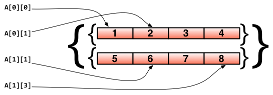#### slice

The main disadvantage of arrays is that we need to know the size prehand. At times this isn't possible. For this, we need a "dynamic array". This is called a `slice` in Go.

`slice` is not really a `dynamic array`. It's a reference type. `slice` points to an underlying `array` whose declaration is similar to `array`, but doesn't need length.

``````// just like defining an array, but this time, we exclude the length.
var fslice []int
``````

Then we define a `slice`, and initialize its data.

``````slice := []byte {'a', 'b', 'c', 'd'}
``````

`slice` can redefine existing slices or arrays. `slice` uses `array[i:j]` to slice, where `i` is the start index and `j` is end index, but notice that `array[j]` will not be sliced since the length of the slice is `j - i`.

``````// define an array with 10 elements whose types are bytes
var ar = byte {'a', 'b', 'c', 'd', 'e', 'f', 'g', 'h', 'i', 'j'}

// define two slices with type []byte
var a, b []byte

// 'a' points to elements from 3rd to 5th in array ar.
a = ar[2:5]
// now 'a' has elements ar,ar and ar

// 'b' is another slice of array ar
b = ar[3:5]
// now 'b' has elements ar and ar
``````

Notice the differences between `slice` and `array` when you define them. We use `[…]` to let Go calculate length but use `[]` to define slice only. Slices do not have length, slices point to arrays which have lengths.

Their underlying data structure.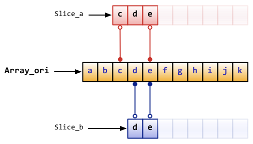slice has some convenient operations.

• `slice` is 0-based, `ar[:n]` equals to `ar[0:n]`
• The second index will be the length of `slice` if omitted, `ar[n:]` equals to `ar[n:len(ar)]`.
• You can use `ar[:]` to slice whole array, reasons are explained in first two statements.

More examples:

``````// define an array
var array = byte{'a', 'b', 'c', 'd', 'e', 'f', 'g', 'h', 'i', 'j'}
// define two slices
var aSlice, bSlice []byte

// some convenient operations
aSlice = array[:3] // equals to aSlice = array[0:3] aSlice has elements a,b,c
aSlice = array[5:] // equals to aSlice = array[5:10] aSlice has elements f,g,h,i,j
aSlice = array[:]  // equals to aSlice = array[0:10] aSlice has all elements

// slice from slice
aSlice = array[3:7]  // aSlice has elements d,e,f,g，len=4，cap=7
bSlice = aSlice[1:3] // bSlice contains aSlice, aSlice, so it has elements e,f
bSlice = aSlice[:3]  // bSlice contains aSlice, aSlice, aSlice, so it has d,e,f
bSlice = aSlice[0:5] // slice could be expanded in range of cap, now bSlice contains d,e,f,g,h
bSlice = aSlice[:]   // bSlice has same elements as aSlice does, which are d,e,f,g
``````

`slice` is a reference type, so any changes will affect other variables pointing to the same slice or array. For instance, in the case of `aSlice` and `bSlice` above, if you change the value of an element in `aSlice`, `bSlice` will be changed as well.

`slice` is like a struct by definition and it contains 3 parts.

• A pointer that points to where `slice` starts.
• The length of `slice`.
• Capacity, the length from start index to end index of `slice`.
``````Array_a := byte{'a', 'b', 'c', 'd', 'e', 'f', 'g', 'h', 'i', 'j'}
Slice_a := Array_a[2:5]
``````

The underlying data structure of the code above as follows.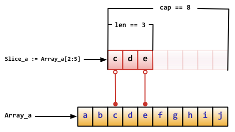There are some built-in functions for slice.

• `len` gets the length of `slice`.
• `cap` gets the maximum length of `slice`
• `append` appends one or more elements to `slice`, and returns `slice` .
• `copy` copies elements from one slice to the other, and returns the number of elements that were copied.

Attention: `append` will change the array that `slice` points to, and affect other slices that point to the same array.

Also, if there is not enough length for the slice (`(cap-len) == 0`), `append` returns a new array for this slice. When this happens, other slices pointing to the old array will not be affected.

#### map

Map is a key value pair, like a dictionary in Python. Use the form `map[keyType]valueType` to define it.

The 'set' and 'get' values in `map` are similar to `slice`, however the index in `slice` can only be of type 'int' while `map` can use much more than that: for example `int`, `string`, or whatever you want. Also, they are all able to use `==` and `!=` to compare values.

``````// use string as the key type, int as the value type, and `make` initialize it.
var numbers map[string] int
// another way to define map
numbers := make(map[string]int)
numbers["one"] = 1  // assign value by key
numbers["ten"] = 10
numbers["three"] = 3

fmt.Println("The third number is: ", numbers["three"]) // get values
// It prints: The third number is: 3
``````

Some notes when you use map.

• `map` is disorderly. Everytime you print `map` you will get different results. It's impossible to get values by `index`. You have to use `key`.
• `map` doesn't have a fixed length. It's a reference type just like `slice`.
• `len` works for `map` also. It returns how many `key`s that map has.
• It's quite easy to change the value through `map`. Simply use `numbers["one"]=11` to change the value of `key` one to `11`.

You can use form `key:val` to initialize map's values, and `map` has built-in methods to check if the `key` exists.

Use `delete` to delete an element in `map`.

``````// Initialize a map
rating := map[string]float32 {"C":5, "Go":4.5, "Python":4.5, "C++":2 }
// map has two return values. For the second return value, if the key doesn't
//exist，'ok' returns false. It returns true otherwise.
csharpRating, ok := rating["C#"]
if ok {
fmt.Println("C# is in the map and its rating is ", csharpRating)
} else {
fmt.Println("We have no rating associated with C# in the map")
}

delete(rating, "C")  // delete element with key "c"
``````

As I said above, `map` is a reference type. If two `map`s point to same underlying data, any change will affect both of them.

``````m := make(map[string]string)
m["Hello"] = "Bonjour"
m1 := m
m1["Hello"] = "Salut"  // now the value of m["Hello"] is Salut
``````

#### make, new

`make` does memory allocation for built-in models, such as `map`, `slice`, and `channel`, while `new` is for types' memory allocation.

`new(T)` allocates zero-value to type `T`'s memory, returns its memory address, which is the value of type `*T`. By Go's definition, it returns a pointer which points to type `T`'s zero-value.

`new` returns pointers.

The built-in function `make(T, args)` has different purposes than `new(T)`. `make` can be used for `slice`, `map`, and `channel`, and returns a type `T` with an initial value. The reason for doing this is because the underlying data of these three types must be initialized before they point to them. For example, a `slice` contains a pointer that points to the underlying `array`, length and capacity. Before these data are initialized, `slice` is `nil`, so for `slice`, `map` and `channel`, `make` initializes their underlying data and assigns some suitable values.

`make` returns non-zero values.

The following picture shows how `new` and `make` are different.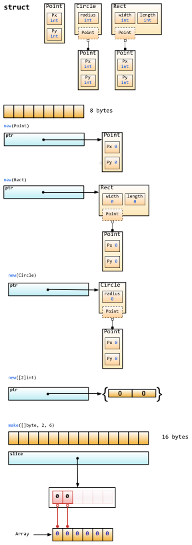Zero-value does not mean empty value. It's the value that variables default to in most cases. Here is a list of some zero-values.

``````int     0
int8    0
int32   0
int64   0
uint    0x0
rune    0 // the actual type of rune is int32
byte    0x0 // the actual type of byte is uint8
float32 0 // length is 4 byte
float64 0 //length is 8 byte
bool    false
string  ""
``````